## Latest Post

### Chemical Equilibrium Questions and Answers

Given,
 (i) H2 (g) + 1/2 S2 (g) = H2S (g), KP1 (ii) 2H2 (g) + S2 (g) = 2H2S (g), KP2
Show that, KP2 = (KP1)2
For the first reaction, ΔG10 = - RT lnKP1
For the second reaction, ΔG20 = - RT lnKP2
Since, ΔG20 = 2ΔG10, therefore, it follows that,
- RT lnKP2 = - 2RT lnKP1
 ∴ KP2 = (KP1)2Equilibrium Constant
Use Gibbs - Helmholtz equation to derive the Vant Hoff reaction isochore. Under What condition do you expect a linear relationship between logk and 1/T ?
The Gibbs - Helmholtz equation is,
ΔG0 = ΔH0 + T[d(ΔG0)/dT]P
Zero superscript is indicating the stranded values.
or, - (ΔH0/T2 ) = -(ΔG0/T2 ) + 1/T[d(ΔG0)/dT]P
or, - (ΔH0/T2 ) = [d/dT(ΔG0/T)]P
Again Vant Hoff isotherm is,
- RT lnKP = ΔG0
or, - R lnKP = ΔG0/T
Differentiating with respect to T at constant P,
- R [dlnKP/dT]P = [d/dT(ΔG0/T)]P
Comparing the above two equation we have,
 dlnKP/dT = (ΔH0/T2
This is the Van't Hoff Equation isochore.
Greater the value of ΔH0, the faster the equilibrium constant(KP)changes with temperature(T), ΔH0 should remains constant for the linear plot of logKP vs 1/T.
How does the equilibrium constant for a reaction, 2A + 3B 4C + Heat, Change when (i) pressure is increases (ii) Temperature is decreases (iii) a catalyst added?
1. Equilibrium constant remains same when P is increases .
2. The reaction is exothermic, hence ΔH = (-)ve so the equilibrium constant is increased with decreases of temperature.
3. Equilibrium constant remains same though a catalyst is added.
4. ΔG0 = - RT lnKa but ΔG0 is not changed due to addition of catalyst as the latter does not participate in the reaction. The initial state and the final state of a chemical reaction remains same weather the catalyst is added or not.
Hence the equilibrium constant (Ka) remains unchanged.
For a reaction,2A + B 2C, ΔG0(500 K) = 2 KJ mol-1. Find the KP at 500 K for the reaction A + ½B C.
ΔG0 (500 K) for the reaction,
A + ½B C
= (2 KJ mol-1)/2
= 1 KJ mol-1
The relation is, ΔG0 = - RT lnKP
or, 1 = - (8.31 × 10-3) × (500) lnKP
or, lnKP = 1/(8.31 × 0.5)
= 0.2406
KP = 1.27
Justify or criticize the following: "The Equilibrium yield of products can not change if the equilibrium constant is kept fixed."
This statement is not correct.
Equilibrium yield of the product is changed if pressure is changed (Δγ ≠ 0), if inert gas is added at constant P (Δγ ≠ 0), and any of the reacting component is added a depleted.
For example, N2 + 3 H2 2NH3
Equilibrium yield of NH3 is increased if P is increased though equilibrium constant kept fixed.
Justify or criticize the following: Heat of reaction is the same weather a catalyst is used or not.
H is a state function hence ΔH (heat of a reaction) does no change if initial state and final state of a Process is same.
A catalyst can not change the initial and final state of a chemical reaction, hence ΔH remains same weather a catalyst is used or not.
Therefore the statement is correct.

## Method of Balancing Oxidation - Reduction Reactions:

Oxidation and reduction are always found to go hand in hand during a redox reaction. Whenever an element or compound is oxidized, another element or another compound must be reduced. An oxidant is reduced and simultaneously the reductant is oxidized.It follows from our above discussion that we should be able to balance oxidation reduction reactions on the basis of
(1) Ion - Electron Method of Balancing Oxidation - Reduction Reactions.
(2) Oxidation Number Method of Balancing Oxidation - Reduction Reactions.

### 1. Ion - Electron Method of Balancing Oxidation - Reduction Reactions:

(i) Ascertain the Reactants and Products, and their chemical formulas.
(ii) Set up the partial equations representing the reduction of the oxidant, and the oxidation of the reductant.
(iii) If the reaction occurs in acid medium use requisite number of H⁺ for balancing the number of atoms involved in the partial equation. for alkaline medium use OH⁻ ions.
(iv) Balancing the charge in the partial equations by adding suitable number of electrons. These electrons indicate the electrons involves in the oxidation or the reduction half reactions.
(v) Multiply each partial equation by a suitable factor so each of the two partial equations involves the same number of electrons.
(vi) Add the partial equations and cancel out species which appear on both sides of the equations.
Some examples will now needed to elucidate the rules.
 In aqueous acid medium potassium permanganate oxidizes a ferrous ion to the ferric state.
In this reaction permanganate ion is the oxidant and ferrous ion the reductant.
The left hand side of the ultimate equation will carry, MnO₄⁻ (KMnO₄), H⁺ (H₂SO₄) and Fe²⁺ (FeSO₄).
The Right hand side will have as products, Mn²⁺ (MnSO₄), H₂O and Fe³⁺ [Fe₂(SO₄)₃].
The partial equation representing the reduction of the oxidant MnO₄⁻ will involve,
MnO₄⁻ Mn²⁺
Since the reaction occurs in acid medium we utilize eight H⁺ ions to balance the four oxygen atoms in MnO₄⁻.
Thus,  MnO₄⁻+8H⁺ → Mn²⁺+4H₂O
Since the above partial equation is still unbalanced from the viewpoint of charge, the equa-tion is balanced by bringing in five electrons:
 MnO₄⁻ + 8H⁺ + 5e ⇆ Mn²⁺ + 4H₂O
Note that MnO₄⁻ Mn²⁺ is indeed a five electron reduction, Mn⁷⁺ + 5e Mn²⁺.
If we now apply the above steps to obtain the partial equation representing the oxidation of reductant we have the following:
 Fe⁺² ⇆ Fe⁺³ + e
This equation on multiplication by 5 and then adding to the partial equation of the oxidant gives the final balanced equation:
 MnO₄⁻ + 8H⁺ + 5e ⇆ Mn²⁺ + 4H₂O 5× ( Fe⁺² ⇆ Fe⁺³ + e)
 MnO₄⁻+8H⁺+5Fe⁺²→Mn²⁺+5Fe⁺³+4H₂O
 The Oxidation of potassium iodide by potassium dichromate in dilute acid medium.
In this reaction dichromate is reduced to (+6) state to (+3) state and iodide ion is oxidised to elementary iodine.
Taking dichromate side, balancing the atoms and charges provides the partial equation:
Cr₂O₇⁻² + 14H⁺ + 6e 2Cr⁺³ + 7H₂O
(Reduction of Oxidant)
Considering the case of iodide ion we get:
2I⁻ I₂ + 2e
(Oxidation of Reductant)
Multiplying the above equation by 3 and adding these two partial equation we have the following final equation:
 Cr₂O₇⁻² + 14H⁺ + 6e ⇆ 2Cr⁺³ + 7H₂O 3 (2I⁻ ⇆ I₂ + 2e) Cr₂O₇⁻²+14H⁺+6I⁻→2Cr⁺³+3I₂+7H₂O

Express by ion electron method the reduction of permagnate to manganous stat by hydrogen peroxide in acid medium.

This Reaction occurs in acid medium and the partial equation is:
 MnO₄⁻ + 8H⁺ + 5e ⇆ Mn⁺² + 4H₂O (Reduction of Oxidant)
In acid medium H₂O₂ will give O₂ and the partial equation being:
 H₂O₂ ⇆ 2H⁺ + O₂ +2e  (Oxidation of Reductant)
First equation is multiplying by 2 and the second is 5 to have electron balanced.
We have,
 2MnO₄⁻ + 16H⁺ + 10e ⇆ 2Mn⁺² + 8H₂O 5H₂O₂ ⇆ 10H⁺ + 5O₂ +10e 2MnO₄⁻+5H₂O₂+6H⁺⇆2Mn⁺²+8H₂O+5O₂
 The reaction of permanganate ion with Sodium Stannite (Na₂SnO₂) in alkaline solution in which manganese dioxide (MnO₂) and Sodium Stannate(Na₂SnO₃) are formed.
The Partial equation representing the reduction of oxidant is,
MnO₄⁻  MnO₂.
Since the medium is alkaline we put the requisite number of OH⁻ ions to effect atom balance as:
MnO₄⁻ + 2H₂O MnO₂ + 4OH⁻
To balance the charge on both sides three electrons are added on the left hand side:
MnO₄⁻ + 2H₂O + 3e  MnO₂ + 4OH⁻
For the reductant the balance partial equation is:
SnO₂⁻² + OH⁻ SnO₃⁻² + H₂O + 2e
To balance the number of electrons involved in the two partial equations the first equation is multiplied by 2 and the second by 3 and on the adding we have the final form of the ionic equation:
2MnO₄⁻ + 4H₂O + 6e  2MnO₂ + 8OH⁻
3SnO₂⁻² + 3OH⁻  3SnO₃⁻² + 3H₂O + 6e

2MnO₄⁻+3SnO₂⁻²+H₂O2MnO₂+3SnO₃⁻²+2OH⁻
Oxidation of Mn⁺² to MnO₄⁻ by PbO₂ or NaBiO₃ in Acid Medium:Representation Oxidation Reduction Reaction

Express by ion - electron method the reduction of nitrate ion to
ammonia by aluminium in aqueous NaOH.

In alkaline medium we use H₂O and OH⁻ ion according to convenience. The partial equation with charge balance is:
NO₃⁻ + 6H₂O + 8e NH₃ + 9OH⁻
Metallic aluminium will go over to aluminate ion, the partial equation being:
Al + 4OH⁻ AlO₂⁻ + 2H₂O +3e
Multiplying by right factors for electron balance we have the balanced equation:
3NO₃⁻ + 18H₂O + 24e 3NH₃ + 27OH⁻
8Al + 32OH⁻ 8AlO₂⁻ + 16H₂O +24e

3NO₃⁻+8Al+2H₂O+5OH⁻3NH₃+8AlO₂⁻

### Oxidation Number Method of Balancing Oxidation - Reduction Reactions:

(i) Assuming that the oxidation number of oxygen remains unchanged manganese in MnO₄⁻ has an oxidation number +7. Calculation of the decrease and increase in oxidation number gives:Representation of Decreases and Increases ofOxidation Number
Putting right factors the decreases and increases in oxidation numbers are balanced, giving,
MnO₄⁻ + 5Fe⁺² Mn⁺² + 5Fe⁺³
Since the reaction medium is acidic and the changes are not equal on the two sides of the above expression, H⁺ ions are added and requisite number of H₂O written:
MnO₄⁻+8H⁺+5Fe⁺²Mn⁺²+ 5Fe⁺³+4H₂O

Use Oxidation Number method to balance the reaction of iodide ion
and iodate ion in acid medium to liberate iodine.

The reaction represented as, I⁻ + IO₃⁻ I₂
Representation of the above equation with oxidation number of iodine,
I⁻(-1) + IO₃⁻(+5) I₂(0)
Since I⁻ (-1) oxidation number of iodide increases by 1 and IO₃⁻(+5) oxidation number decreases by 5.
Putting the right factors the decrease and increase in oxidation number balanced:
5I⁻ + IO₃⁻ 3I₂
Since the medium is acidic and the charges are unequal on two sides of the above expression we put Six H⁺ and write three H₂O to balanced the equation:
I⁻ + IO₃⁻ + 6H⁺ I₂ + 3H₂O
 The Oxidation of iodide ion by dichromate ion in acid medium:
The reaction represented as,
Cr₂O₇⁻ + I⁻ Cr⁺³ + I₂The Oxidation of Iodide ion by dichromateion in Acid medium
 Oxidation of sodium stannite to stannate in alkaline medium:
This reaction represented as,
MnO₄⁻ + SnO₂⁻² MnO₂ + SnO₃⁻²
Oxidation number of Mn decreases by 3 and oxidation number of Sn increases by 2.
Equalizing the increase and decrease in oxidation number,
2MnO₄⁻ + 3SnO₂⁻² 2MnO₂ + 3SnO₃⁻²
Because the medium is alkaline and because the ionic charges are not equal on the two sides, two OH⁻ ions are added to the right hand side.
2MnO₄⁻+3SnO₂⁻²2MnO₂+3SnO₃⁻²+2OH⁻
Since there are two hydrogen atoms on the right and none on the left, H₂O is added to make atom balance. The final equation is:
2MnO₄⁻+3SnO₂⁻²+H₂O2MnO₂+3SnO₃⁻²+2OH⁻

Use oxidation number method to balance the reaction between
Sulphurus acid and dichromate in acidic medium.

The reaction represented as,
SO₃⁻² + Cr₂O₇⁻² SO₄⁻² + 2Cr⁺³
Oxidation of two Cr decreases by 2 × (+3) = 6 and oxidation number of S increases by 2.
Equalizing the above equation increase and decrees in oxidation number,
We have, 3SO₃⁻² + Cr₂O₇⁻²  3SO₄⁻² + 2Cr⁺³
Because of the medium is acidic and the ionic charges are not equal on the two sides, eight H⁺ ions are added to the Left hand side.
3SO₃⁻² + Cr₂O₇⁻² + 8H⁺  3SO₄⁻² + 2Cr⁺³
Since there are eight hydrogen ion on the left and none on the right, four H₂O added to make atom balance.
Thus the final equation is:
3SO₃⁻²+Cr₂O₇⁻²+8H⁺3SO₄⁻²+2Cr⁺³+4H₂O

## How to Calculate Dipole Moment of a Compound?

### (i) For Penta-atomic Molecules(AX₄):

The molecules of this type CH₄, CCl₄, PtCl₄ are the examples of having zero dipole moment. This suggests that they are either regular tetrahedral or square planer. But polar molecules of this type have pyramidal structures.
Let us discuss the structure of CH₄ that have regular tetrahedral structure and the angle of each H-C-H is 109°28ˊ.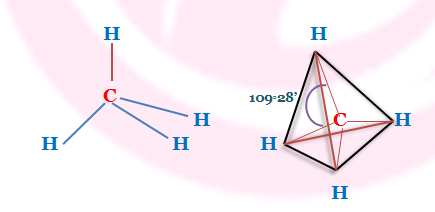Structure of CH₄
The electric moment associated with a group is called group moment and it depends on the arrangement of the bonds in the group and the difference of the electronegativity of the constituent atoms forming the bonds in the group.
It can be shown that the group moment of CH₃ (mCH₃) is identical with the bond moment of C-H (mC-H) and so the two moments cancel each other resulting zero dipole moment of the molecule in CH₄.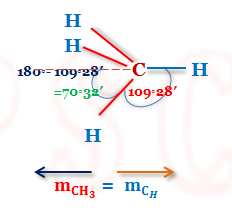Group Moment of CH₄ Molecule
 Thus , mCH₃ = 3 mCH Cos(180° -109°28՛)  = 3 mCH Cos 70°32՛  = 3 mCH × (1/3) = mCH

#### Another method to show that resultant moment of CH₄ is zero: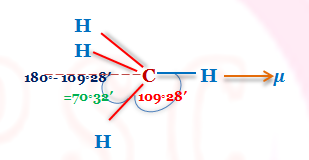Resultant Dipole Moment of Methane
 μ = mCH (1 + 3 Cos 109°28՛)  = mCH {1 - (3 ×(1/3)} = 0
By similar calculation, it can be shown that mCCl3 = mClCCl4

This idea explains the same value of μ for CH₃Cl and CHCl₃.

 Thus, μ² = m12 + m22 + 2 m1m2 Cosθ But here θ = 0° hence Cosθ = 1 ∴ μ² = m12 + m22 + 2 m1m2 = (m1 + m2)² or, μ = (m1 + m2)  =  (mCCl + mCH)  = (1.5 D + 0.4 D) = 1.9 D
 μ = (m1 + m2)  =  (mCH + mCCl) = (0.4 D + 1.5 D)  = 1.9 D

Similar calculation can done for the group moment of C₂H₄, C₃H₇, C₄H₉ etc. have the same vale and equal to the bond moment of C-H. The identical value of dipole moment of the homologous alcohols and saturated hydrocarbons confirms the above statement.

### (ii) Hexa Atomic Molecules( AX₅):

The molecules of this type are PCl₅, AsCl₅, PF₅ etc have μ =0 indicating that they have pyramidal structure having center of symmetry.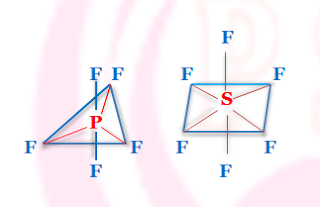Structure of PF₅ and SF₆

### (iii) Hepta-Atomic Molecules( AX₅):

Hepta-atomic molecules like SF₆, XeF₆, WF₆ etc have μ =0 indicating that these have octahedral symmetrical structure.

### (iv) Benzene and its Derivatives:

Zero dipole moment of the benzene suggested that it has regular hexagonal planer structure and it confirms Kekule’s form.Regular Planer Hexagonal Structure ofBenzene having Centre of Symmetry
However, if hydrogen atom is substituted by another atom or group, it acquire polar character.
Examples of such derivative of benzene are C₆H₅Cl, C₆H₅NO₂, C₆H₅OH etc.
When di-substituted benzene are considered it can be shown that o-isomer will have highest value of dipole moment then other two isomers. p-derivative has lowest value while m-derivative has the value between two.
The values can be calculated using the vector addition principle
μ = m12 + m22 + 2 m1m2 cosθ
provided the group must also be lie on the same plane of the benzene ring.

### Di-substituted derivative of benzene: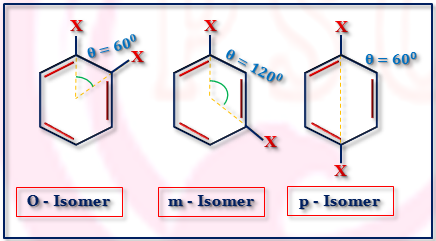Di-substituted derivative of benzene
 μ² = m12 + m22 + 2 m1m2 cosθ Here, m1 = m2 = m and θ = 60° Thus, μ² = 2 m²(1 + cos60°)  = 2 m² (1 + 1/2)  ∴ μ= √3 m
 μ² = m12 + m22 + 2 m1m2 cosθ Here, m1 = m₂ = m and θ = 120° Thus, μ² = 2 m²(1 + cos120°)  = 2 m² (1 - 1/2) ∴ μ = m
 μ² = m12 + m22 + 2 m1m₂ cosθ Here, m1 = m2= m and θ = 180° Thus, μ² = 2 m²(1 + cos180°)  = 2 m² (1 - 1) ∴ μ = 0
Thus μ of o-isomer〉
μ of m-isomer〉μ of p-isomer
(this has been confirmed for C₆H₄Cl₂ and C₆H₄(NO₂)₂.
This determination of dipole moment helps to determine the orientation of the groups in the benzene ring.
p - dinitro benzene has μ = 0 but p - dihydroxy benzene μ ≠ 0. Explain.
Through the p-dinitro benzene has μ = 0. p-dihydroxy benzene has dipole moment(μ ≠ 0). This is due to the fact that the two substituted hydroxy group are not on the same plane of the benzene ring but are inclined to the ring.

### (v) Cis and Trans forms of Geometrical Isomerism:

Presence of double bond in C - C link restricts the free rotation and geometrical isomerism develops.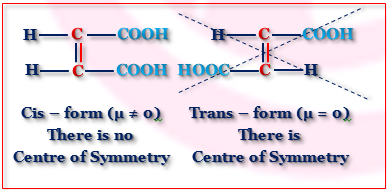Dipole Moment of Cis and Trans Isomers
The Measurement of dipole moment helps to distinguish the two forms of geometrical isomers.

### (vi) Change of Dipole Moment with Temperature:

For molecule CH₂Cl - CH₂Cl, several conformations are also changed and so dipole moment (μ) of the molecule is also changed.

The dipole moment of o-xylene = 0.693 D. Find the dipole moment of toluene.
For o - xylene the θ = 60° and μ = √3 m. Thus, m = 0.693/√3 = 0.4 D
Again θ = 120° for toluene and dipole moment(μ) of toluene = m.
Thus the dipole moment of toluene is 0.4 D.

Name

Email *

Message *